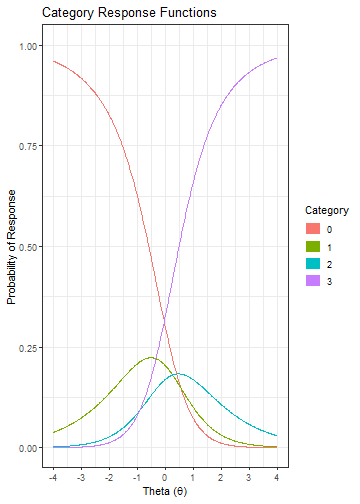This vignettes covers the psychometric models that are implemented in the irt package.

# Item Models

Name Description Parameters
Rasch Rasch Model b
1PL One-Parameter Logistic Model b, D
2PL Two-Parameter Logistic Model a, b, D
3PL Three-Parameter Logistic Model a, b, c, D
4PL Four-Parameter Logistic Model a, b, c, d, D
GRM Graded Response Model a, b, D
PCM Partial Credit Model b
GPCM Generalized Partial Credit Model a, b, D
GPCM2 Reparameterized Generalized Partial Credit Model a, b, d, D

## Rasch Model

For an examinee $$i$$ with ability $$\theta_i$$, the probability of correct response to an item $$j$$ is:

$P\left(X_{ij} = 1 | \theta_i;b_j\right) = \frac{e^{(\theta_i - b_j)}}{1 + e^{(\theta_i - b_j)}}$

where , $$b_j$$ is the item difficulty (or threshold) of item $$j$$.

User needs to specify only the item difficulty parameter:

library(irt)

itm_rasch <- item(b = -1.29)
itm_rasch
#> A 'Rasch' item.
#> Model:   Rasch (Rasch Model)
#> Model Parameters:
#>   b = -1.29
#>
#> --------------------------


The probability of each response option at $$\theta = -.65$$ is:

prob(ip = itm_rasch, theta = -0.65)
#>              0         1
#> [1,] 0.3452465 0.6547535


The item characteristic curve of this item is:

plot(itm_rasch)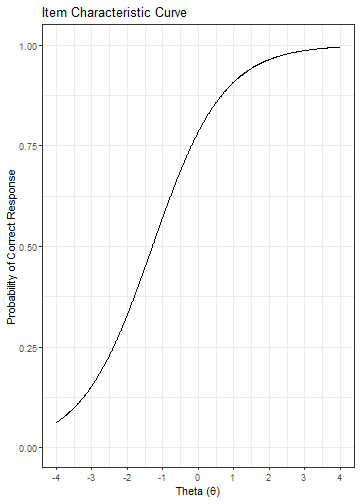## One-Parameter Logistic Model

For an examinee $$i$$ with ability $$\theta_i$$, the probability of correct response to an item $$j$$ is:

$P\left(X_{ij} = 1 | \theta_i;b_j\right) = \frac{e^{D(\theta_i - b_j)}}{1 + e^{D(\theta_i - b_j)}}$

where , $$b_j$$ is the item difficulty (or threshold) of item $$j$$. D is the scaling constant (the default value is 1).

User needs to specify the following parameters:

itm_1pl <- item(b = 0.83, D = 1)
itm_1pl
#> A '1PL' item.
#> Model:   1PL (One-Parameter Logistic Model)
#> Model Parameters:
#>   b = 0.83
#>   D = 1
#>
#> --------------------------


The probability of each response option at $$\theta = .73$$ is:

prob(ip = itm_1pl, theta = 0.73)
#>              0         1
#> [1,] 0.5249792 0.4750208


The item characteristic curve of this item is:

plot(itm_1pl)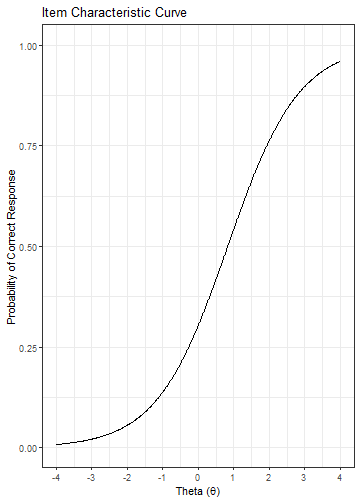## Two-Parameter Logistic Model

For an examinee $$i$$ with ability $$\theta_i$$, the probability of correct response to an item $$j$$ is:

$P\left(X_{ij} = 1 | \theta_i;a_j, b_j\right) = \frac{e^{Da_j(\theta_i - b_j)}}{1 + e^{Da_j(\theta_i - b_j)}}$

where $$a_j$$ is the item discrimination (or slope) of item $$j$$, $$b_j$$ is the item difficulty (or threshold). D is the scaling constant (the default value is 1).

User needs to specify all of the parameter values:

itm_2pl <- item(a = .94, b = -1.302, D = 1)
itm_2pl
#> A '2PL' item.
#> Model:   2PL (Two-Parameter Logistic Model)
#> Model Parameters:
#>   a = 0.94
#>   b = -1.302
#>   D = 1
#>
#> --------------------------


The probability of each response option at $$\theta = -0.53$$ is:

prob(ip = itm_2pl, theta = -0.53)
#>              0         1
#> [1,] 0.3261434 0.6738566


The item characteristic curve of this item is:

plot(itm_2pl)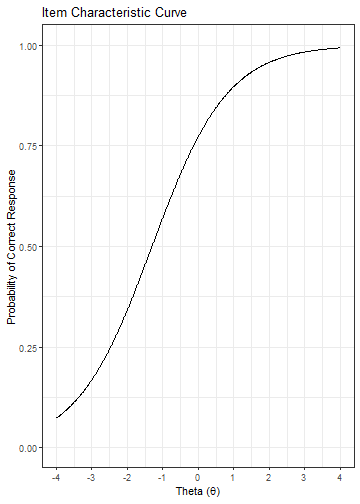## Three-Parameter Logistic Model

For an examinee $$i$$ with ability $$\theta_i$$, the probability of correct response to an item $$j$$ is:

$P\left(X_{ij} = 1 | \theta_i;a_j, b_j, c_j\right) = c_j + (1 - c_j)\frac{e^{Da_j(\theta_i - b_j)}}{1 + e^{Da_j(\theta_i - b_j)}}$

where $$a_j$$ is the item discrimination (or slope) of item $$j$$, $$b_j$$ is the item difficulty (or threshold), and $$c_j$$ is the lower asymptote (or pseudo-guessing) value. D is the scaling constant (the default value is 1).

As can be seen from the equation above, the user needs to specify all of the parameter values:

itm_3pl <- item(a = 1.51, b = 2.04, c = .16, D = 1.7)
itm_3pl
#> A '3PL' item.
#> Model:   3PL (Three-Parameter Logistic Model)
#> Model Parameters:
#>   a = 1.51
#>   b = 2.04
#>   c = 0.16
#>   D = 1.7
#>
#> --------------------------


The probability of each response option at $$\theta = 1.5$$ is:

prob(ip = itm_3pl, theta = 1.5)
#>              0         1
#> [1,] 0.6719846 0.3280154


The item characteristic curve of this item:

plot(itm_3pl)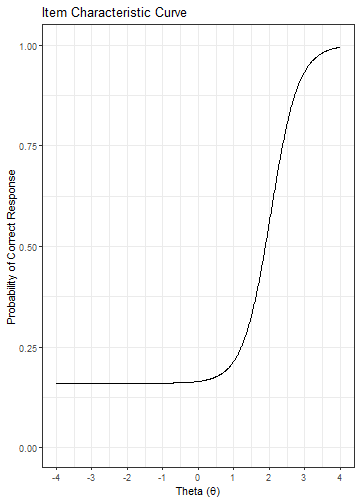## Four-Parameter Logistic Model

For an examinee $$i$$ with ability $$\theta_i$$, the probability of correct response to an item $$j$$ is:

$P\left(X_{ij} = 1 | \theta_i;a_j, b_j, c_j, d_j\right) = c_j + (d_j - c_j)\frac{e^{Da_j(\theta_i - b_j)}}{1 + e^{Da_j(\theta_i - b_j)}}$

where $$a_j$$ is the item discrimination (or slope) of item $$j$$, $$b_j$$ is the item difficulty (or threshold), $$c_j$$ is the lower asymptote (or pseudo-guessing) value and $$d_j$$ is the upper asymptote. D is the scaling constant (the default value is 1).

As can be seen from the equation above, the user needs to specify all of the parameter values:

itm_4pl <- item(a = 1.2, b = -.74, c = .22, d = .99, D = 1.7)
itm_4pl
#> A '4PL' item.
#> Model:   4PL (Four-Parameter Logistic Model)
#> Model Parameters:
#>   a = 1.2
#>   b = -0.74
#>   c = 0.22
#>   d = 0.99
#>   D = 1.7
#>
#> --------------------------


The probability of each response option at $$\theta = 1.2$$ is:

prob(ip = itm_4pl, theta = 1.2)
#>               0        1
#> [1,] 0.02443797 0.975562


The item characteristic curve of this item is:

plot(itm_4pl)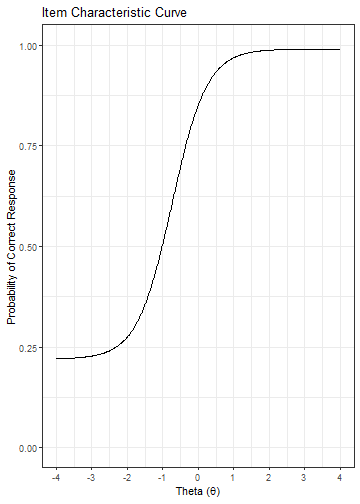For an examinee $$i$$ with ability $$\theta_i$$, the probability of responding at or above the category $$k$$ to an item $$j$$ with possible scores $$k = 0, 1, \ldots, m_j$$:

$P^*\left(X_{ij} = k | \theta_i;a_j, b_j\right) = \frac{e^{Da_j(\theta_i - b_{jk})} }{1 + e^{Da_j(\theta_i - b_{jk})}}$

where $$a_j$$ is the item discrimination (or slope) of item $$j$$, $$b_{jk}$$ is the threshold parameter. Note that the probability of responding at or above the lowest category is $$P^*\left(X_{ij} = 0\right) = 1$$. Responding at a category $$k$$ can be calculated as:

$P(X_{ij}=k|\theta_i) = P^*(X_{ij} = k) - P^*(X_{ij} = k+1)$

The user needs to specify the following parameter values:

itm_grm <- item(a = 0.84, b = c(-1, -.2, .75, 1.78), D = 1.7, model = "GRM")
itm_grm
#> A 'GRM' item.
#> Model:   GRM (Graded Response Model)
#> Model Parameters:
#>   a = 0.84
#>   b = -1;  -0.2;  0.75;  1.78
#>   D = 1.7
#>
#> --------------------------


The probability of each response option at $$\theta = 1.13$$ is:

prob(ip = itm_grm, theta = 1.13)
#>               0          1         2         3       4
#> [1,] 0.04557977 0.08461474 0.2373791 0.3491363 0.28329


The option characteristic curves of this item is:

plot(itm_grm)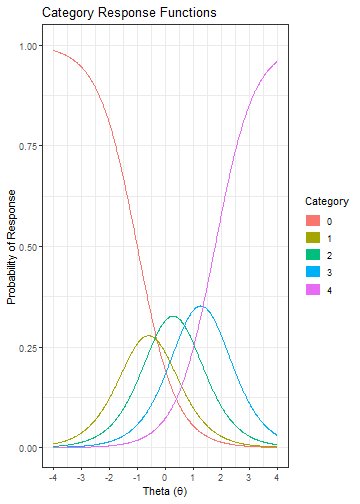## Generalized Partial Credit Model (GPCM)

For an examinee $$i$$ with ability $$\theta_i$$, the probability of a response $$k$$ to an item $$j$$ with possible scores $$k = 0, 1, \ldots, m_j$$:

$P\left(X_{ij} = k | \theta_i;a_j, b_j\right) = \frac{\text{exp} \left( \sum_{v = 0}^{k}Da_j(\theta_i - b_{jv}) \right) } {{\sum_{h = 0}^{m_j} \text{exp} \left[ \sum_{v = 0}^{h} Da_j(\theta_i - b_{jv}) \right] }}$

where $$a_j$$ is the item discrimination (or slope) of item $$j$$, $$b_{jv}$$ are the step difficulty parameters. Note that $$b_{jv}$$ values are not necessarily ordered from smallest to the largest. D is the scaling constant (the default value is 1). $$\sum_{v = 0}^0 Da_j(\theta_i - b_{jv}) = 0$$.

The user needs to specify the following parameter values:

itm_gpcm <- item(a = 1.1, b = c(-.74, .3, .91, 2.19), D = 1.7, model = "GPCM")
itm_gpcm
#> A 'GPCM' item.
#> Model:   GPCM (Generalized Partial Credit Model)
#> Model Parameters:
#>   a = 1.1
#>   b = -0.74;  0.3;  0.91;  2.19
#>   D = 1.7
#>
#> --------------------------


The probability of each response option at $$\theta = -0.53$$ is:

prob(ip = itm_gpcm, theta = -0.53)
#>              0         1         2           3            4
#> [1,] 0.3551121 0.5259117 0.1113895 0.007540084 4.659945e-05


The option characteristic curves of this item is:

plot(itm_gpcm)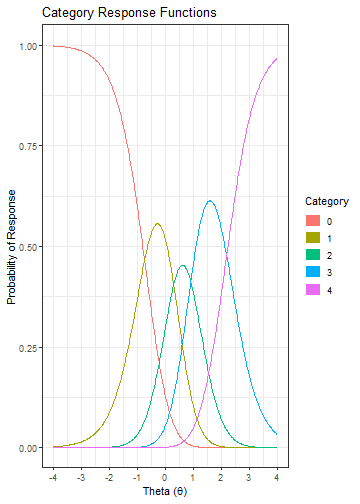## Partial Credit Model (PCM)

For an examinee $$i$$ with ability $$\theta_i$$, the probability of a
response $$k$$ to an item $$j$$ with possible scores $$k = 0, 1, \ldots, m_j$$:

$P\left(X_{ij} = k | \theta_i;b_j\right) = \frac{\text{exp} \left( \sum_{v = 0}^{k}(\theta_i - b_{jv}) \right) } {{\sum_{h = 0}^{m_j} \text{exp} \left[ \sum_{v = 0}^{h} (\theta_i - b_{jv}) \right] }}$

where $$b_{jv}$$ are the step difficulty parameters. $$\sum_{v = 0}^0 (\theta_i - b_{jv}) = 0$$.

The user needs to specify the following parameter values:

itm_pcm <- item(b = c(-1.38, -.18, 1.1), model = "PCM")
itm_pcm
#> A 'PCM' item.
#> Model:   PCM (Partial Credit Model)
#> Model Parameters:
#>   b = -1.38;  -0.18;  1.1
#>
#> --------------------------


The probability of each response option at $$\theta = -1.09$$ is:

prob(ip = itm_pcm, theta = -1.09)
#>              0         1         2          3
#> [1,] 0.3407646 0.4554072 0.1833124 0.02051573


The option characteristic curves of this item is:

plot(itm_pcm)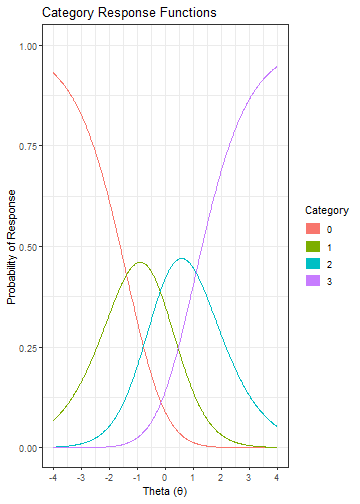## Reparameterized Generalized Partial Credit Model (GPCM2)

For an examinee $$i$$ with ability $$\theta_i$$, the probability of a response $$k$$ to an item $$j$$ with possible scores $$k = 0, 1, \ldots, m_j$$:

$P\left(X_{ij} = k | \theta_i;a_j, b_j, d_j\right) = \frac{\text{exp} \left( \sum_{v = 0}^{k}Da_j(\theta_i - b_j + d_{jv}) \right) } {{\sum_{h = 0}^{m_j} \text{exp} \left[ \sum_{v = 0}^{h} Da_j(\theta_i - b_j + d_{jv}) \right] }}$

where $$a_j$$ is the item discrimination (or slope) of item $$j$$, $$b_j$$ is the overall location parameter and $$d_{jv}$$ are the threshold parameters. D is the scaling constant (the default value is 1).

The user needs to specify the following parameter values:

itm_gpcm2 <- item(a = .71, b = .37, d = c(-.18, .11, 1.29), D = 1,
model = "GPCM2")
itm_gpcm2
#> A 'GPCM2' item.
#> Model:   GPCM2 (Reparameterized Generalized Partial Credit Model)
#> Model Parameters:
#>   a = 0.71
#>   b = 0.37
#>   d = -0.18;  0.11;  1.29
#>   D = 1
#>
#> --------------------------


The probability of each response option at $$\theta 1.3$$ is:

prob(ip = itm_gpcm2, theta = 1.3)
#>               0          1       2         3
#> [1,] 0.04254421 0.07246065 0.15163 0.7333651


The option characteristic curves of this item is:

plot(itm_gpcm2)